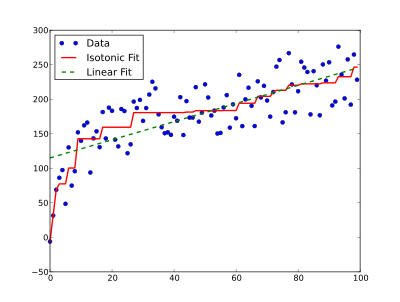# Isotonic regressionAn example of isotonic regression (solid red line) compared to linear regression on the same data, both fit to minimize the mean squared error. The free-form property of isotonic regression means the line can be steeper where the data are steeper; the isotonicity constraint means the line does not decrease.

In statistics, isotonic regression or monotonic regression is the technique of fitting a free-form line to a sequence of observations under the following constraints: the fitted free-form line has to be non-decreasing everywhere, and it has to lie as close to the observations as possible.

## Applications

Isotonic regression has applications in statistical inference, for example, to fit of an isotonic curve to mean experimental results when an order is expected. A benefit of isotonic regression is that it does not assume any form for the target function, such as linearity assumed by linear regression.

Another application is nonmetric multidimensional scaling, where a low-dimensional embedding for data points is sought such that order of distances between points in the embedding matches order of dissimilarity between points. Isotonic regression is used iteratively to fit ideal distances to preserve relative dissimilarity order.

Software for computing isotone (monotonic) regression has been developed for the R statistical package  and the Python programming language.

## Algorithms

In terms of numerical analysis, isotonic regression involves finding a weighted least-squares fitto a vectorwith weights vectorsubject to a set of non-contradictory constraints of the kind. The usual choice for the constraints is, or in other words: every point must be at least as high as the previous point.

Such constraints define a partial ordering or total ordering and can be represented as a directed graph, where N is the set of variables (observed values) involved, and E is the set of pairs (i, j) for each constraint. Thus, the IR problem corresponds to the following quadratic program (QP):In the case whenis a total ordering, a simple iterative algorithm for solving this quadratic program is called the pool adjacent violators algorithm. Conversely, Best and Chakravarti (1990) studied the problem as an active set identification problem, and proposed a primal algorithm. These two algorithms can be seen as each other's dual, and both have a computational complexity of O(n).

## Simply ordered case

To illustrate the above, let theconstraints be.

The isotonic estimator,, minimizes the weighted least squares-like condition:whereis the set of all piecewise linear, non-decreasing, continuous functions andis a known function.

1. Kruskal, J. B. (1964). "Nonmetric Multidimensional Scaling: A numerical method". Psychometrika. 29 (2): 115–129. doi:10.1007/BF02289694.
2. Leeuw, Jan de; Hornik, Kurt; Mair, Patrick (2009). "Isotone Optimization in R: Pool-Adjacent-Violators Algorithm (PAVA) and Active Set Methods". Journal of Statistical Software. 32 (5): 1–24. doi:10.18637/jss.v032.i05. ISSN 1548-7660.
3. Best, M.J.; Chakravarti N. (1990). "Active set algorithms for isotonic regression; a unifying framework". Mathematical Programming. 47: 425–439. doi:10.1007/BF01580873.
• Robertson, T.; Wright, F. T.; Dykstra, R. L. (1988). Order restricted statistical inference. New York: Wiley. ISBN 0-471-91787-7.
• Barlow, R. E.; Bartholomew, D. J.; Bremner, J. M.; Brunk, H. D. (1972). Statistical inference under order restrictions; the theory and application of isotonic regression. New York: Wiley. ISBN 0-471-04970-0.
• Shively, T.S., Sager, T.W., Walker, S.G. (2009). "A Bayesian approach to non-parametric monotone function estimation". Journal of the Royal Statistical Society, Series B. 71 (1): 159–175. doi:10.1111/j.1467-9868.2008.00677.x.
• Wu, W. B.; Woodroofe, M.; Mentz, G. (2001). "Isotonic regression: Another look at the changepoint problem". Biometrika. 88 (3): 793–804. doi:10.1093/biomet/88.3.793.